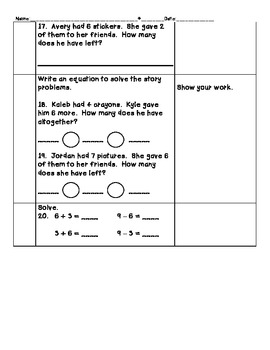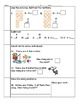# Subtraction Pre-test for Common CoreSubject
Grade Levels
Resource Type
Product Rating
File Type

PDF (Acrobat) Document File

Be sure that you have an application to open this file type before downloading and/or purchasing.

817 KB|3 pages
Share
Product Description
Supports CCSS:
- 1.OA.1. Use addition and subtraction within 20 to solve word problems involving situations of adding to, taking from, putting together, taking apart, and comparing, with unknowns in all positions, e.g., by using objects, drawings, and equations with a symbol for the unknown number to represent the problem.
-1.OA.3. Apply properties of operations as strategies to add and subtract.
-1.OA.4. Understand subtraction as an unknown-addend problem.
-1.OA.5. Relate counting to addition and subtraction (e.g., by counting on 2 to add 2).
-1.OA.6. Add and subtract within 20, demonstrating fluency for addition and subtraction within 10. Use strategies such as counting on; making ten (e.g., 8 + 6 = 8 + 2 + 4 = 10 + 4 =
-1.OA.8. Determine the unknown whole number in an addition or subtraction equation relating to three whole numbers.

Questions require students to:
-Solve subtraction equation. Cross out the picture to match
the problem.
-Subtract by crossing out the picture. Then write the equation.
-Use pictures to subract
-Subtract horizontally and vertically
-Count on to solve and check.
--Differentiate between and solve addition and subtraction story problems.
--Write equations for subtraction problems
-Solve fact families
Total Pages
3 pages
Answer Key
N/A
Teaching Duration
N/A
Report this ResourceSign Up